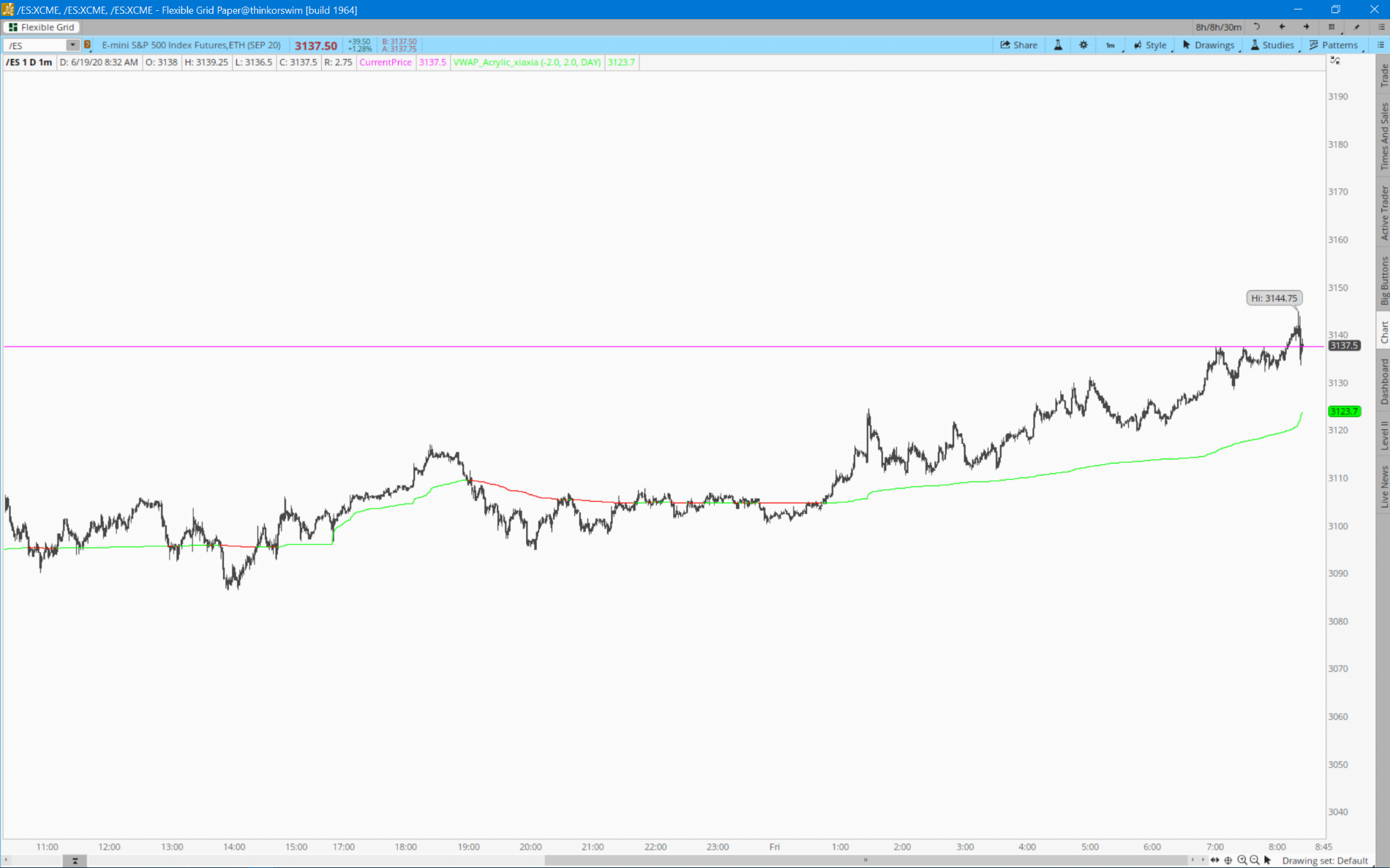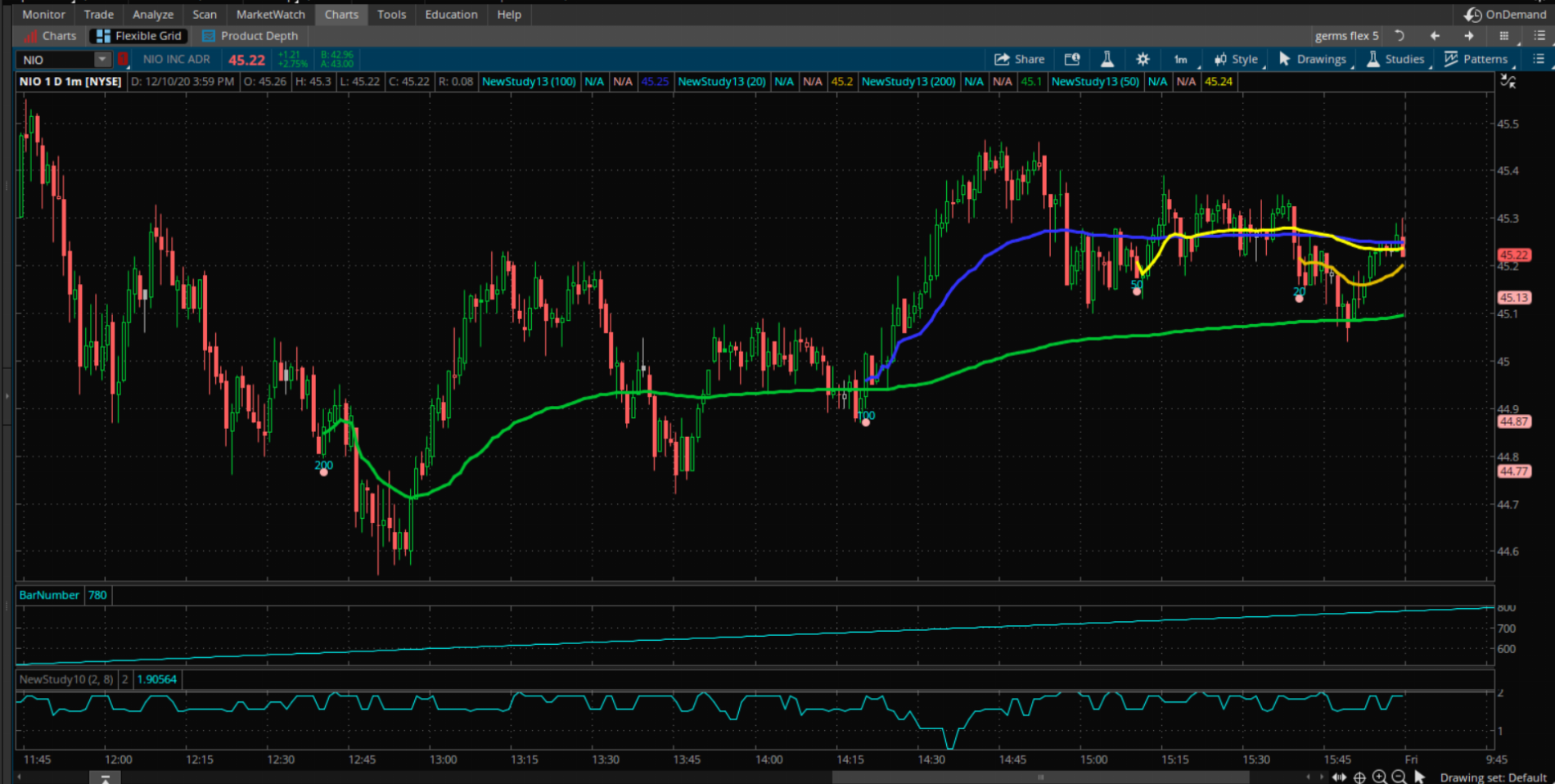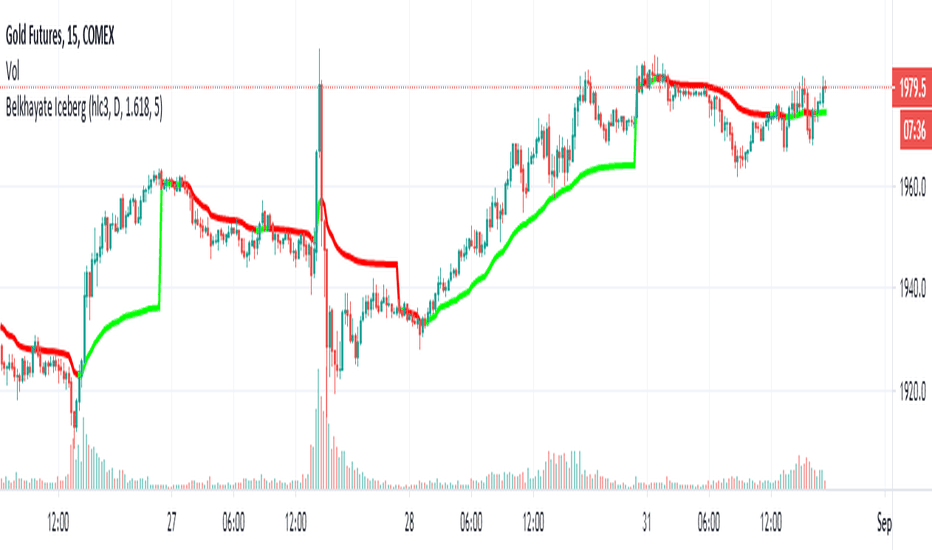# Xiaxia's Acrylic VWAP Indicator for ThinkorSwim

#### xiaxia

##### New member
Howdy there partners, just made a simple alteration to the classic VWAP.
• When stock is trading above VWAP, the VWAP line will be green.
• When stock is trading below VWAP, the VWAP line will be red.
love you all and happy tradingCode:
``````input numDevDn = -2.0;
input numDevUp = 2.0;
input timeFrame = {default DAY, WEEK, MONTH};

def cap = getAggregationPeriod();
def errorInAggregation =
timeFrame == timeFrame.DAY and cap >= AggregationPeriod.WEEK or
timeFrame == timeFrame.WEEK and cap >= AggregationPeriod.MONTH;
assert(!errorInAggregation, "timeFrame should be not less than current chart aggregation period");

def yyyyMmDd = getYyyyMmDd();
def periodIndx;
switch (timeFrame) {
case DAY:
periodIndx = yyyyMmDd;
case WEEK:
periodIndx = Floor((daysFromDate(first(yyyyMmDd)) + getDayOfWeek(first(yyyyMmDd))) / 7);
case MONTH:
periodIndx = roundDown(yyyyMmDd / 100, 0);
}
def isPeriodRolled = compoundValue(1, periodIndx != periodIndx, yes);

def volumeSum;
def volumeVwapSum;
def volumeVwap2Sum;

if (isPeriodRolled) {
volumeSum = volume;
volumeVwapSum = volume * vwap;
volumeVwap2Sum = volume * Sqr(vwap);
} else {
volumeSum = compoundValue(1, volumeSum + volume, volume);
volumeVwapSum = compoundValue(1, volumeVwapSum + volume * vwap, volume * vwap);
volumeVwap2Sum = compoundValue(1, volumeVwap2Sum + volume * Sqr(vwap), volume * Sqr(vwap));
}
def price = volumeVwapSum / volumeSum;
def deviation = Sqrt(Max(volumeVwap2Sum / volumeSum - Sqr(price), 0));

plot VWAP = price;
plot UpperBand = price + numDevUp * deviation;
plot LowerBand = price + numDevDn * deviation;

VWAP.setDefaultColor(getColor(0));
VWAP.AssignValueColor(if close>=VWAP then Color.Green else Color.Red);
UpperBand.setDefaultColor(getColor(2));
LowerBand.setDefaultColor(getColor(4));``````Last edited:
very good, thanks

very good, thanks

I was excited to try being big fan of VWAP but didn’t work. It is exactly same as normal VWAP.

@monicasgupta That's correct, this is just a modified version of the regular VWAP. All it does is change the color of the VWAP line to green when price is trading above or red when price is below VWAP.

thank BenTen, i didn’t observe the color change after adding this vwap.

Looking for a basic coloring of the candles above / below the VWAP line.

@Alex I assume green if above VWAP and red if below it? If so, use the code below:

Code:
``````# Modified VWAP
# TD Ameritrade IP Company, Inc. (c) 2011-2020
#

input numDevDn = -2.0;
input numDevUp = 2.0;
input timeFrame = {default DAY, WEEK, MONTH};

def cap = getAggregationPeriod();
def errorInAggregation =
timeFrame == timeFrame.DAY and cap >= AggregationPeriod.WEEK or
timeFrame == timeFrame.WEEK and cap >= AggregationPeriod.MONTH;
assert(!errorInAggregation, "timeFrame should be not less than current chart aggregation period");

def yyyyMmDd = getYyyyMmDd();
def periodIndx;
switch (timeFrame) {
case DAY:
periodIndx = yyyyMmDd;
case WEEK:
periodIndx = Floor((daysFromDate(first(yyyyMmDd)) + getDayOfWeek(first(yyyyMmDd))) / 7);
case MONTH:
periodIndx = roundDown(yyyyMmDd / 100, 0);
}
def isPeriodRolled = compoundValue(1, periodIndx != periodIndx, yes);

def volumeSum;
def volumeVwapSum;
def volumeVwap2Sum;

if (isPeriodRolled) {
volumeSum = volume;
volumeVwapSum = volume * vwap;
volumeVwap2Sum = volume * Sqr(vwap);
} else {
volumeSum = compoundValue(1, volumeSum + volume, volume);
volumeVwapSum = compoundValue(1, volumeVwapSum + volume * vwap, volume * vwap);
volumeVwap2Sum = compoundValue(1, volumeVwap2Sum + volume * Sqr(vwap), volume * Sqr(vwap));
}
def price = volumeVwapSum / volumeSum;
def deviation = Sqrt(Max(volumeVwap2Sum / volumeSum - Sqr(price), 0));

plot VWAP = price;
plot UpperBand = price + numDevUp * deviation;
plot LowerBand = price + numDevDn * deviation;

VWAP.setDefaultColor(getColor(0));
UpperBand.setDefaultColor(getColor(2));
LowerBand.setDefaultColor(getColor(4));

AssignPriceColor(if close > VWAP then color.green else color.red);``````

•Rrm411, Beltrame1 and Alex
I'm trying to add a condition to this to only turn green if above vwap. thanks for the help.

Code:
``````input price = close;
input fastLength = 9;
input slowLength = 20;
input averageType = AverageType.EXPONENTIAL;

plot FastMA = MovingAverage(averageType, price, fastLength);
plot SlowMA = MovingAverage(averageType, price, slowLength);
FastMA.AssignValueColor(if FastMA > SlowMA then color.green else color.red);
SlowMA.AssignValueColor(if FastMA > SlowMA then color.green else color.red);

plot ArrowUp = if FastMA crosses above SlowMA
then low
else double.nan;
ArrowUP.SetPaintingStrategy(PaintingStrategy.Arrow_UP);
ArrowUP.SetLineWeight(3);
ArrowUP.SetDefaultColor(Color.Green);

plot ArrowDN = if FastMA crosses below SlowMA
then high
else double.nan;
ArrowDN.SetPaintingStrategy(PaintingStrategy.Arrow_DOWN);
ArrowDN.SetLineWeight(3);
ArrowDN.SetDefaultColor(Color.Red);

def countUP = if !isNaN(ArrowUp)
then 1
else if FastMA > SlowMA
then countUP + 1
else if !isNaN(ArrowDN)
then 0
else countUP;
def countDN = if !isNaN(ArrowDN)
then 1
else if FastMA < SlowMA
then countDN + 1
else if !isNaN(ArrowUP)
then 0
else countDN;
AddLabel(1, "Count UP = " + countUP, color.white);
AddLabel(1, "Count DN = " + countDN, color.white);
def CrossBar = if FastMA crosses SlowMa
then barNumber()
else double.nan;

input length = 50;

plot Vol = volume;
plot VolAvg = Average(volume, length);

Vol.SetPaintingStrategy(PaintingStrategy.HISTOGRAM);
Vol.SetLineWeight(3);
Vol.DefineColor("Up", Color.UPTICK);
Vol.DefineColor("Down", Color.DOWNTICK);
Vol.AssignValueColor(if close > close then Vol.Color("Up") else if close < close then Vol.Color("Down") else GetColor(1));
VolAvg.SetDefaultColor(GetColor(8));

def HAopen;
def HAhigh;
def HAlow;
def HAclose;
HAopen = CompoundValue(1, (haopen + haclose) / 2, (open + close) / 2);
HAhigh = Max(high, close);
HAlow = Min(low, close);
haclose = (HAopen + HAclose + HAlow + close) / 4;
AssignPriceColor(if HAclose > HAopen
then color.green
else color.red);

AssignBackgroundColor( if Fastma > slowma and HAclose < HAopen and close > close then Vol.Color("Up") else Color.CURRENT);

Alert(if Fastma > slowma and HAclose < HAopen and close > close then 1 else 0, " ", Alert.Bar, Sound.ring);``````

Does anyone have the Vwap where the price above is green and below it's red?

Does anyone have the Vwap where the price above is green and below it's red?
this gives you alot of things, it paints clouds if its aboce and below

Code:
``````# study name: TheVWAP_Intraday
# author:     © Zachary M. Hurwitz
# date:       August 11, 2017
#
# email:      [EMAIL][email protected][/EMAIL]
# website:    [URL]https://www.theVWAP.com[/URL]

#hint StDev1: Standard deviations from VWAP (primary band).
#hint StDev2: Standard deviations from VWAP (secondary band).
#hint timeframe: Day (resetting at market open), Week (resetting on the first day of the week), or Month (resetting on the first day of the month).
#hint VWAP_Color: SlopeBasic plots either a gold (upsloping) or pink (downsloping) VWAP curve. \n </li> SlopeSignal plots a blue VWAP curve based on VWAP slope for trend up, pink for trend down, and white for undetermined direction. \n </li>Single plots a monochromatic (blue) VWAP curve.
#hint Background_Color_Signal: <li><b>Cloud:</b> displays the Green/Red dynamic background colorization depending on position relative to VWAP. \n <li><b>Fast_Lane:</b> displays highlighting of 'fast lane' (surpassing +/- 1.0 standard deviations from VWAP) price action. \n <li><b>Both:</b> displays both.  Obviously. \n <li><b>None:</b> displays neither.  Duh. </li>
#hint Show_Dev_Signal: Displays the secondary band (StDev2) Green/Red colorization depending on proximity/position relative to VWAP.
#hint Tolerance_Band_Width: Standard deviation distance of tolerance bands around the primary (StDev1) curve.  Default is 0; any value > 0 will enable the tolerance bands (ex: 0.1, 0.25, etc., expressed in StDev).
#hint High_or_Low_of_x_bars: Number of bars of which the current bar must be a high (or low) in order to qualify as a flagged/identified extreme.  Default is 0 (i.e., off) but recommended setting is ~30-60m for intraday trading (and thus, 30-60 bars for 1m charts or 6-12 bars for 5m charts, depending on user preference).

input StDev1 = 1.0;
input StDev2 = 2.0;
input timeFrame = {default DAY, WEEK, MONTH};
input VWAP_Color = {default SlopeBasic, SlopeSignal, Single};

input Background_Color_Signal = {default Cloud, Fast_Lane, Both, None};
def show_cloud = if background_color_signal == background_color_signal."Cloud" or background_color_signal == background_color_signal."Both" then 1 else 0;
def show_fast_lane = if background_color_signal == background_color_signal."Fast_Lane" or background_color_signal == background_color_signal."Both" then 1 else 0;

input Show_Dev_Signal = no;
input Tolerance_Band_Width = 0.0;
def ToleranceTrue = Tolerance_Band_Width > 0.0;

input High_or_Low_of_x_bars = 0;
def high_low_threshold = 0.25;
def HLBarLength = if High_or_Low_of_x_bars == 0 then Double.NaN else High_or_Low_of_x_bars;

def Show_Day_Divider = Yes;
def Equities_Futures = if ticksize() * tickvalue() == 0.0001 then 1 else 0;
def tooearly = 0;
#if Equities_Futures and GetTime() <= Market_Open + 1200000 then 1 else 0;
def cap = GetAggregationPeriod();
def errorInAggregation = timeFrame == timeFrame.DAY and cap >= AggregationPeriod.WEEK or timeFrame == timeFrame.WEEK and cap >= AggregationPeriod.MONTH;
def yyyyMmDd = GetYYYYMMDD();

def periodIndx;
switch (timeFrame) {
case DAY:
periodIndx = yyyyMmDd;
case WEEK:
periodIndx = Floor((DaysFromDate(First(yyyyMmDd)) + GetDayOfWeek(First(yyyyMmDd))) / 7);
case MONTH:
periodIndx = RoundDown(yyyyMmDd / 100, 0);
}
def NewDay = CompoundValue(1, periodIndx != periodIndx, yes);
def highestDays = TotalSum(NewDay);

rec VolumeSum;
rec MoneySum;
rec volumeVwap2Sum;

if (NewDay) {
VolumeSum = volume;
MoneySum = volume * vwap;
volumeVwap2Sum = volume * Sqr(vwap);
} else {
VolumeSum = CompoundValue(1, VolumeSum + volume, volume);
MoneySum = CompoundValue(1, MoneySum + volume * vwap, volume * vwap);
volumeVwap2Sum = CompoundValue(1, volumeVwap2Sum + volume * Sqr(vwap), volume * Sqr(vwap));
}

def VW = MoneySum / VolumeSum;
def deviation = Sqrt(Max(volumeVwap2Sum / VolumeSum - Sqr(VW), 0));

def slopelength = 5;
def slopelookback = 8;
def slope = ((VW - VW[slopelength]) / slopelength) / close * 100000;

def slopeup = if slope[slopelookback] > 1 then 1 else 0;
def slopedn = if slope[slopelookback] < -1 then 1 else 0;
def nonslope = if !slopeup and !slopedn then 1 else 0;

def v_counter_low = fold index = 1 to 90 with v_tempvar = 0 while v_tempvar == 0 do if low[index] < low then index else 0;
def v_counter_high = fold index2 = 1 to 90 with v_tempvar2 = 0 while v_tempvar2 == 0 do if high[index2] > high then index2 else 0;

plot VWAP;
plot UpperBand_2;
plot LowerBand_2;
plot UpperBand_1;
plot LowerBand_1;
plot OuterBand_H;
plot InnerBand_H;
plot InnerBand_L;
plot OuterBand_L;

if (!errorInAggregation) {
VWAP = VW;
UpperBand_2 = VW + StDev2 * deviation;
LowerBand_2 = VW - StDev2 * deviation;
UpperBand_1 = VW + StDev1 * deviation;
LowerBand_1 = VW - StDev1 * deviation;
OuterBand_H = VW + ((1 + Tolerance_Band_Width) * deviation);
InnerBand_H = VW + ((1 - Tolerance_Band_Width) * deviation);
InnerBand_L = VW - ((1 - Tolerance_Band_Width) * deviation);
OuterBand_L = VW - ((1 + Tolerance_Band_Width) * deviation);
} else {
VWAP = Double.NaN;
UpperBand_2 = Double.NaN;
LowerBand_2 = Double.NaN;
UpperBand_1 = Double.NaN;
LowerBand_1 = Double.NaN;
OuterBand_H = Double.NaN;
InnerBand_H = Double.NaN;
InnerBand_L = Double.NaN;
OuterBand_L = Double.NaN;
}

UpperBand_2.AssignValueColor(if tooearly then Color.DARK_GRAY else if Show_Dev_Signal and slope >= 0 and slopeup then Color.GREEN else Color.GRAY);
UpperBand_2.SetDefaultColor(Color.GRAY);
UpperBand_2.HideBubble();
UpperBand_2.HideTitle();

OuterBand_H.SetDefaultColor(Color.GRAY);
OuterBand_H.HideBubble();
OuterBand_H.HideTitle();
OuterBand_H.SetHiding(!ToleranceTrue);

UpperBand_1.AssignValueColor(if tooearly then Color.DARK_GRAY else Color.WHITE);
UpperBand_1.SetDefaultColor(Color.WHITE);
UpperBand_1.SetStyle(Curve.SHORT_DASH);
UpperBand_1.HideBubble();
UpperBand_1.HideTitle();

InnerBand_H.SetDefaultColor(Color.GRAY);
InnerBand_H.HideBubble();
InnerBand_H.HideTitle();
InnerBand_H.SetHiding(!ToleranceTrue);

VWAP.SetDefaultColor(Color.CYAN);
VWAP.DefineColor("VWAP Ascending", Color.ORANGE);
VWAP.DefineColor("VWAP Descending", Color.MAGENTA);
VWAP.DefineColor("Above VWAP",CreateColor(0,199,145));
VWAP.DefineColor("Below VWAP",Color.DARK_RED);
VWAP.DefineColor("Fast Lane +", Color.CYAN);
VWAP.DefineColor("Fast Lane -",CreateColor(150,0,50));

VWAP.AssignValueColor(if VWAP_Color == VWAP_Color.SlopeSignal and slopeup and slopeup and slopeup and slopeup and slopeup then Color.CYAN
else if VWAP_Color == VWAP_Color.SlopeSignal and slopedn and slopedn and slopedn and slopedn and slopedn then Color.MAGENTA
else if VWAP_Color == VWAP_Color.SlopeBasic and slope > 0 then VWAP.Color("VWAP Ascending")
else if VWAP_Color == VWAP_Color.SlopeBasic and slope <= 0 then VWAP.Color("VWAP Descending")
else if VWAP_Color == VWAP_Color.Single then color.CYAN
else Color.WHITE);

InnerBand_L.SetDefaultColor(Color.GRAY);
InnerBand_L.HideBubble();
InnerBand_L.HideTitle();
InnerBand_L.SetHiding(!ToleranceTrue);

LowerBand_1.AssignValueColor(if tooearly then Color.DARK_GRAY else Color.WHITE);
LowerBand_1.SetDefaultColor(Color.WHITE);
LowerBand_1.SetStyle(Curve.SHORT_DASH);
LowerBand_1.HideBubble();
LowerBand_1.HideTitle();

OuterBand_L.SetDefaultColor(Color.GRAY);
OuterBand_L.HideBubble();
OuterBand_L.HideTitle();
OuterBand_L.SetHiding(!ToleranceTrue);

LowerBand_2.AssignValueColor(if tooearly then Color.DARK_GRAY else if Show_Dev_Signal and slope < 0 and slopedn then Color.RED else Color.GRAY);
LowerBand_2.SetDefaultColor(Color.GRAY);
LowerBand_2.HideBubble();
LowerBand_2.HideTitle();

plot counter_low = if !isNaN(HLBarLength) and v_counter_low > HLBarLength and (between(close,VW-high_low_threshold*deviation,VW+high_low_threshold*deviation) or between(close,VW-(1+high_low_threshold)*deviation,VW-(1-high_low_threshold)*deviation) or between(close,VW+(1-high_low_threshold)*deviation,VW+(1+high_low_threshold)*deviation)) then v_counter_low else Double.NaN;
counter_low.SetPaintingStrategy(paintingstrategy.VALUES_BELOW);
counter_low.SetDefaultColor(color.RED);
counter_low.HideBubble();
counter_low.HideTitle();

plot counter_high = if !isNaN(HLBarLength) and v_counter_high > HLBarLength and (between(close,VW-high_low_threshold*deviation,VW+high_low_threshold*deviation) or between(close,VW-(1+high_low_threshold)*deviation,VW-(1-high_low_threshold)*deviation) or between(close,VW+(1-high_low_threshold)*deviation,VW+(1+high_low_threshold)*deviation)) then v_counter_high else Double.NaN;
counter_high.SetPaintingStrategy(paintingStrategy.VALUES_ABOVE);
counter_high.SetDefaultColor(color.GREEN);
counter_high.HideBubble();
counter_high.HideTitle();

def var_close = if Show_Cloud then close else Double.NaN;
AddCloud(var_close, VW, VWAP.Color("Above VWAP"), VWAP.Color("Below VWAP"));

def var_VWoneup = if Show_Fast_Lane then UpperBand_1 else Double.NaN;
def var_VWonedn = if Show_Fast_Lane then LowerBand_1 else Double.NaN;

AddCloud(close, var_VWonedn, Color.BLACK, VWAP.Color("Fast Lane -"));

AddVerticalLine(if GetAggregationPeriod() < AggregationPeriod.DAY and Show_Day_Divider then if GetTime() == Market_Open and !isNaN(close) then yes else if GetTime() <= Market_Open + 60000 and !isNaN(close) and periodIndx != periodIndx then yes else no else no,"",CreateColor(40,40,40),curve.FIRM);``````

•zeek
Does this also show the previous day's VWAP. Is there script that I could add, if not?

REVERSED ANCHORED VWAP.. instead of setting the vwap and looking forward this will plot the vwap from the current bar and look back the desired number of bars. As price moves so does the vwap.Code:
``````# Identify - Input Bars Back

# Mobius
#German BURRITO

input BarsBack = 50;

def currentBar = if isnan(close[-1]) and !isnan(close)

then barnumber()

else currentBar;

def barCount = if barnumber()== 1

then highestall(currentBar)

else if barCount > 1

then barCount - 1

else 0;

plot count = if barCount == BarsBack then barCount else double.nan;

count.SetPaintingStrategy(PaintingStrategy.Values_Below);

plot Number1 = if barCount == barsBack then low - (3* TickSize()) else double.nan;

Number1.SetStyle(Curve.Points);

Number1.SetLineWeight(5);

Number1.SetDefaultColor(Color.Pink);

def LocH = (hl2) * volume;
def LocL = (hl2) * volume;
rec PH;
rec VH;

if count {
PH = LocH;
VH = volume;
} else {
PH = compoundValue(1, LocH + PH, Double.NaN);
VH = compoundValue(1, volume + VH, Double.NaN);
}

plot Vw =  PH / VH;``````

Hello!

Sending out this request for a short Pinescript code to be converted to thinkscript.
Study("Colored VWAP", overlay=true)
plot( vwap, color=rising(vwap,2)?green:red,style=linebr,linewidth=3,transp=1,title="VWAP")

So essentially what this simple tradingview indicator does is turn green when the current price is above VWAP or turn red if price is below VWAP. Would this be possible to be converted to a TOS study? It would really help with visualization of the price action relative to VWAP.

Thanks!

(see attached image) - just paying attention to the VWAP, it'll either be red or green depending on where the current price is@atomech Add this to the bottom of your vwap script. The pinescript you posted checks if vwap is rising or falling and not if price is above or below vwap.
Code:
``VWAP.AssignValueColor(if close > vwap then color.green else if close < vwap then color.red else color.white);``

•atomech
just paying attention to the VWAP, it'll either be red or green depending on where the current price is

Several versions of labels here, if you'd like to take a peek.

•atomech
thank you both for your help!!

hi any way to shade the area above/below vwap to price? thanks! @BenTen

### Not the exact question you're looking for?

87k+ Posts
162 Online## The Market Trading Game Changer

Join 2,500+ subscribers inside the useThinkScript VIP Membership Club
• Exclusive indicators
• Proven strategies & setups
• Private Discord community
• Exclusive members-only content
• 1 full year of unlimited support

What is useThinkScript?

useThinkScript is the #1 community of stock market investors using indicators and other tools to power their trading strategies. Traders of all skill levels use our forums to learn about scripting and indicators, help each other, and discover new ways to gain an edge in the markets.

How do I get started?

We get it. Our forum can be intimidating, if not overwhelming. With thousands of topics, tens of thousands of posts, our community has created an incredibly deep knowledge base for stock traders. No one can ever exhaust every resource provided on our site.

If you are new, or just looking for guidance, here are some helpful links to get you started.

What are the benefits of VIP Membership?